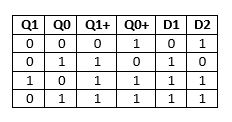# GATE | GATE CS Mock 2018 | Set 2 | Question 57

The next state table of a 2 bit saturating up-counter is given below.The counter is built as synchronous sequential circuit using D flip-flops. The value for D1 and D2 are

(A) D1 = Q0 + Q1
D2 = Q’0 + Q1
(B) D1 = Q’1Q0
D2 = Q’1 + Q’0
(C) D1 = Q’0 + Q1
D2 = Q0 + Q1
(D) None of these

Explanation: Since D = Q, so;Using above excitation table,
D1 = Q0 + Q1
D2 = Q’0 + Q1

Option (A) is correct.

Quiz of this Question

My Personal Notes arrow_drop_up
Article Tags :

Be the First to upvote.

Please write to us at contribute@geeksforgeeks.org to report any issue with the above content.